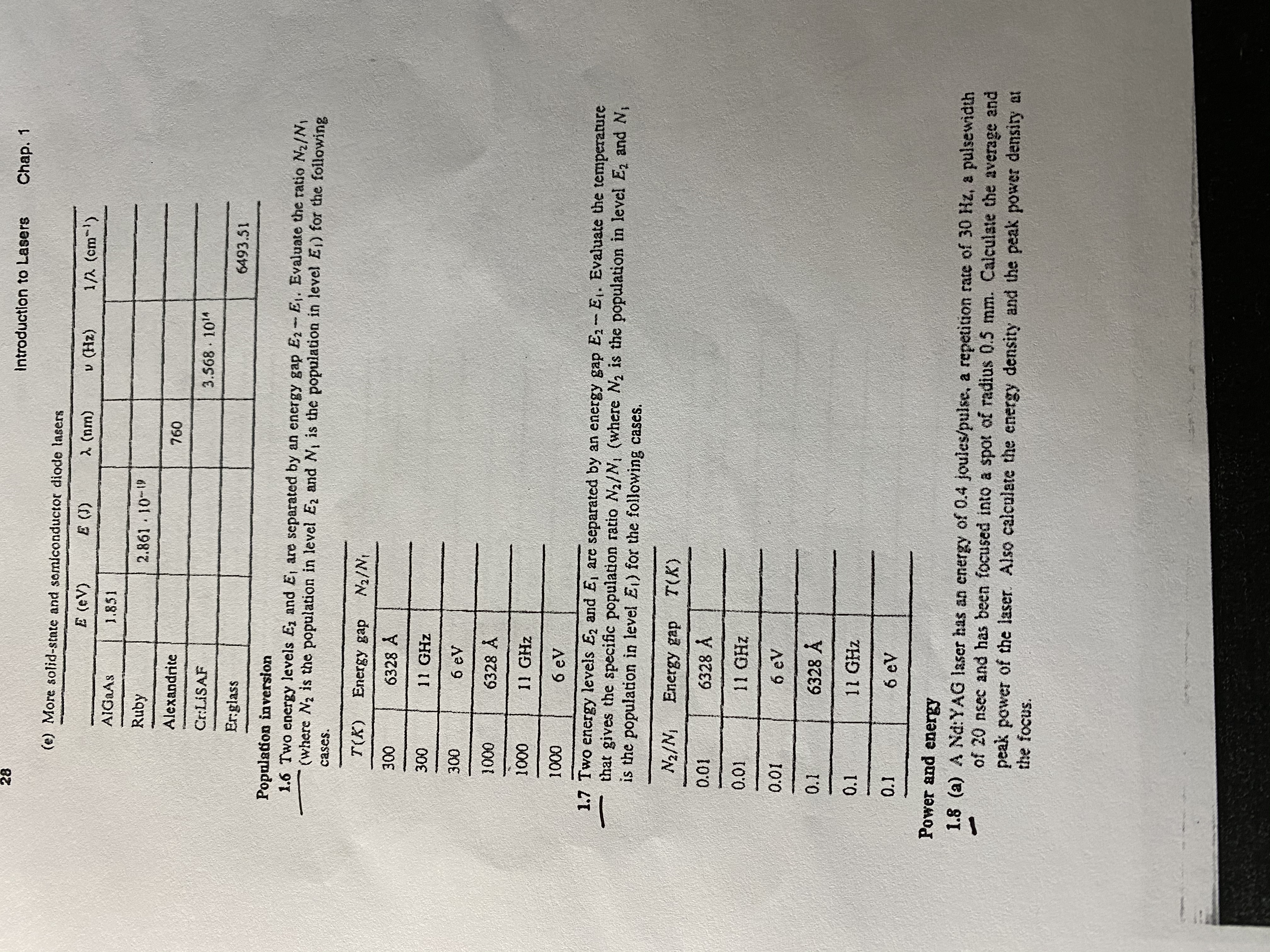# 28Introduction to LasersChap. 1(e) More solid-state and somiconductor diode lasersE (eV)E (J)2 (nm)v (Hz)1/A (cm-)AIGAAS1.851Ruby2.861 10-1Alexandrite760Cr:LISAF3.568 10"Er:glass6493.51Population inversion1.6 Two energy levels E, and E, are separated by an energy gap E2-E,. Evaluate the ratio N2/N,(where N2 is the population in level Ez and N, is the population in level E) for the followingcases.T(K) Energy gapN2/N3006328 A30011 GHz3006 eV10006328 Å100011 GHz10006 eV1.7 Two energy levels E2 and E, are separated by an energy gap E- E. Evaluate the temperaturethat gives the specific population ratio N2/N, (where N2 is the population in level E, and N,is the population in level E) for the following cases.N2/NEnergy gapT(K)0.016328 A0.0111 GHz0.016 eV0.16328 A0.111 GHz0.16 eVPower and energy1.8 (a) A Nd:YAG laser has an energy of 0.4 joules/pulse, a repetition rate of 30 Hz, a pulsewidthof 20 nsec and has been focused into a spot of radius 0.5 mm. Calculste the average andpeak power of the laser. Also calculate the energy density and the peak power density atthe focus.

Question
8 views

1.7help_outlineImage Transcriptionclose28 Introduction to Lasers Chap. 1 (e) More solid-state and somiconductor diode lasers E (eV) E (J) 2 (nm) v (Hz) 1/A (cm-) AIGAAS 1.851 Ruby 2.861 10-1 Alexandrite 760 Cr:LISAF 3.568 10" Er:glass 6493.51 Population inversion 1.6 Two energy levels E, and E, are separated by an energy gap E2-E,. Evaluate the ratio N2/N, (where N2 is the population in level Ez and N, is the population in level E) for the following cases. T(K) Energy gap N2/N 300 6328 A 300 11 GHz 300 6 eV 1000 6328 Å 1000 11 GHz 1000 6 eV 1.7 Two energy levels E2 and E, are separated by an energy gap E- E. Evaluate the temperature that gives the specific population ratio N2/N, (where N2 is the population in level E, and N, is the population in level E) for the following cases. N2/N Energy gap T(K) 0.01 6328 A 0.01 11 GHz 0.01 6 eV 0.1 6328 A 0.1 11 GHz 0.1 6 eV Power and energy 1.8 (a) A Nd:YAG laser has an energy of 0.4 joules/pulse, a repetition rate of 30 Hz, a pulsewidth of 20 nsec and has been focused into a spot of radius 0.5 mm. Calculste the average and peak power of the laser. Also calculate the energy density and the peak power density at the focus. fullscreen
check_circle

Step 1

Energy gap is given by,

Step 2

Population inversion is governed ...

### Want to see the full answer?

See Solution

#### Want to see this answer and more?

Solutions are written by subject experts who are available 24/7. Questions are typically answered within 1 hour.*

See Solution
*Response times may vary by subject and question.
Tagged in

### Science